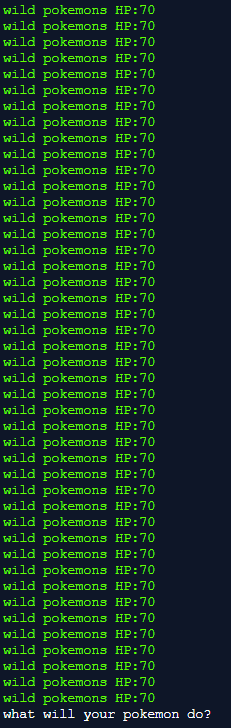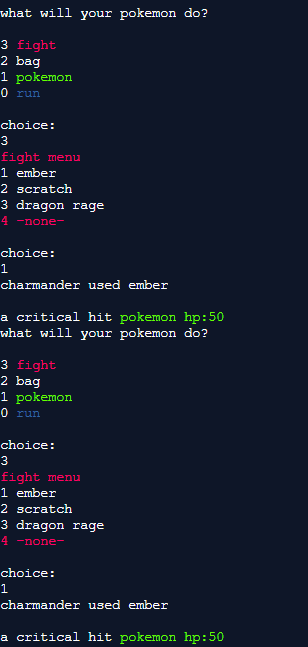← Back to all posts
this is related to a question i asked before and still trying to find an answer that works.

full program: https://repl.it/@Nintenerd7/FIGHT-sequenceN7

(for the past few days i've been trying to find a way to reroll to inflict more damage and i dont want the message to be shown twice before i for back to the battle menu.)

(if i add a for loop in before the switch statement say i wanted it to repeat 9 times it would show the same message 9 times then )( if i don't include it it shows the message only once but once i do the whole fight process all over again it shows something like this)is there any way i can do this without getting those outputs.

language: C++;

\snippet of functioning code.//

#include "Game.h"
#include "colormod.h"
#include <stdlib.h>
using namespace std;
//colours
Color::Modifier red(Color::FG_RED);
Color::Modifier def(Color::FG_DEFAULT);
Color::Modifier green(Color::FG_GREEN);
Color::Modifier blue(Color::FG_BLUE);

Game::Game(){
//press functions
PressEnter = " ";
//

pokemonSelect = 0;
//

//switch pokemon
pokemonSelected = 0;
//

//fighting pokemons hp
partyHP = 100;
//

//level
level = 5;
ExpPoints = 40;
//

//exp
exp = 50;
//

//choices
choiceMain = 0;
choiceThree = 0;
choiceTwo = 0;
choiceOne = 0;
choiceZero = 0;
//

//move
move = 0;
moveTwo = 0;
moveThree = 0;
moveFour = 0;
//

//wild pokemon hp
wPhP = 90;
wPhPtotal =0;
//

//under maitenence message
wip ="";
//

//get playing
playing = true;
//

}

Game::~Game(){

}

//functions

//random
srand(time(NULL));
//

//re-roll
int roll = 999;
//

//dice random
//wild pokemon generator
int pokemonName = 3;
int wildpokemonResult = 0;
wildpokemonResult = rand() % pokemonName +1;

//attack chance
int attackNum = 3;
int attackResult;
attackResult = rand() % attackNum +1;

//pokemon exp bonus
int expBonus = 2;
int expResult = 0;
expResult = rand() % expBonus + 1;
system ("PAUSE");
//END OF dice random

//pokemon encounter at random
switch(wildpokemonResult){
case 1: cout<<green<< " a wild pidgey has appeared"<<" "<<"hp:"<<wPhP<<def<<endl;
break;

case 2: cout<<green<< " a wild rattata has appeared"<<" "<<"hp:"<<wPhP<<def<<endl;
break;

case 3: cout<<green<< " a wild caterpie has appeared"<<" "<<"hp:"<<wPhP<<def<<endl;
break;
}//END OF pokemon encounter at random

cout<< "1 charmander"<<endl;
cout<< "2 squirtle"<<endl;
cout<< "3 bulbasaur"<<endl;
cout<< "4 pikachu"<<endl;

cout<<endl<<"choice: "<<endl;
cin>> pokemonSelect;
//pokemon party

//sending out a pokemon

if (pokemonSelect == 1){
cout<<red<<"go charmander!"<<endl;
cout<<" "<<endl;

}
else if (pokemonSelect == 2){
cout<<red<<"go squirtle!"<<def<<endl;
cout<<" "<<endl;
}
else if (pokemonSelect == 3){
cout<<red<<"go bulbasaur!"<<def<<endl;
cout<<" "<<endl;
}
else if (pokemonSelect == 4){
cout<<red<<"go pikachu!"<<def<<endl;
cout<<" "<<endl;

}//sending out a pokemon

//BATTLE START!
for(int i=1; i<=999; i++){

cout<<" "<<endl;

cout<<"3"<< " "<<red<<"fight"<<endl;
cout<<def<<"2"<< " "<<def<<"bag"<<endl;
cout<<def<<"1"<< " "<<green<<"pokemon"<<endl;
cout<<def<<"0"<< " "<<blue<<"run"<<endl;

cout<<def<<endl<<"choice: "<<endl;
cin>>choiceMain;

switch(choiceMain){
case 0:
playing = false;
break;

default:

break;
}

//fight
if (choiceMain==3)
{
//charmander
if (pokemonSelect==1){
cout<<def<< "1 ember"<<endl;
cout<< "2 scratch"<<endl;
cout<< "3 dragon rage"<<endl;
cout<<red<< "4 -none-"<<endl;

cout<<def<<endl<<"choice: "<<endl;
cin>> move;
if (move==1){
cout<<"charmander used ember"<<endl;
cout<<" "<<endl;

}

else if (move==2){
cout<<"charmander used scratch"<<endl;
cout<<" "<<endl;

}
else if (move==3){
cout<<"used dragon rage"<<endl;
cout<<" "<<endl;

}
}

//squirtle
if (pokemonSelect == 2){
cout<<def<< "1 watergun"<<endl;
cout<< "2 scratch"<<endl;
cout<< "3 tail whip"<<endl;
cout<<red<< "4 -none-"<<endl;

cout<<def<<endl<<"choice: "<<endl;
cin>> move;
if (move==1){
cout<<"squirtle used watergun"<<endl;
cout<<" "<<endl;

}

else if (move==2){
cout<<"squirtle used scratch"<<endl;
cout<<" "<<endl;

}
else if (move==3){
cout<<"squirtle used tail whip";
cout<<" "<<endl;

}
}

//bulbasaur
if(pokemonSelect==3){
cout<<def<< "1 vine whip"<<endl;
cout<< "2 pound"<<endl;
cout<< "3 razor leaf"<<endl;
cout<<red<< "4 -none-"<<endl;

cout<<def<<endl<<"choice: "<<endl;
cin>> move;
if (move==1){
cout<<"bulbasaur used vine whip"<<endl;
cout<<" "<<endl;

}

else if (move==2){
cout<<"bulbasaur used pound"<<endl;
cout<<" "<<endl;

}
else if (move==3){
cout<<"bulbasaur used tail whip";
cout<<" "<<endl;

}

`` } //end if``

//pikachu
if (pokemonSelect==4){
cout<<def<< "1 thundershock"<<endl;
cout<< "2 tackle"<<endl;
cout<< "3 tail whip"<<endl;
cout<<red<< "4 -none-"<<endl;

cout<<def<<endl<<"choice: "<<endl;
cin>> move;

if (move==1){
cout<<"pikachu used thundershock"<<endl;
cout<<" "<<endl;

}

else if (move ==2){
cout<<"pikachu used tackle"<<endl;
cout<<" "<<endl;

}
else if (move==3){
cout<<"pikachu used tail whip";
cout<<" "<<endl;

}

}

//MESSAGE

if (wPhP ==90){
switch(attackResult){
case 1:

cout<< "a critical hit" <<" " << green<<"pokemon hp:"<<wPhP-40<<endl;
break;

case 2: cout<<"the wild pokemon doged your attack"<<endl;
break;

case 3:

cout<<green<<"wild pokemons HP:"<<wPhP-20<<endl;
break;

}//END SWITCH

}//END IF

}//END IF

}//END for

}//END MAIN